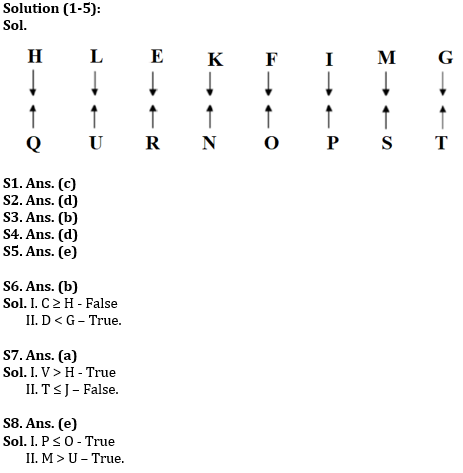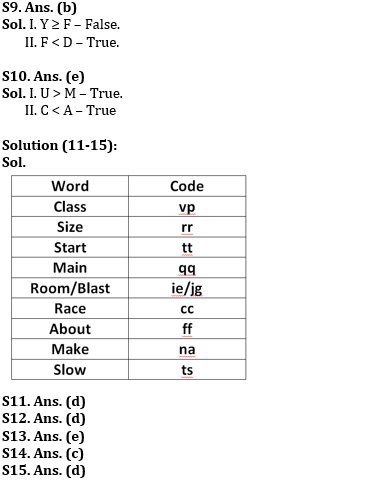Latest Banking jobs   »   Reasoning Ability Quiz For RBI Assistant/...

# Reasoning Ability Quiz For RBI Assistant/ ESIC UDC Mains 2022- 22nd April

Directions (1-5): Study the following information carefully and answer the questions accordingly.
Sixteen people are sitting in two rows. In row 1 – E, F, G, H, I, K, L and M are sitting and facing the south direction and in row 2 – N, O, P, Q, R, S, T and U are sitting and facing the north direction but not necessarily in the same order.
L sits fourth to the right of I. The one who sits opposite to I, sits second to the left of T. The one who sits opposite to L, sits to the right of Q. Two people sit between Q and N who is not opposite to H. Three people sit between G and the one who sits opposite to N. Four people sit between E and G. The one who sits opposite to E, sits three places away from P. F sits second to the right of M who is not opposite to O. R sits immediate right of U.

Q1. Who sits second to the right of O?
(a) G
(b) P
(c) S
(d) T
(e) None of these

Q2. What is the position of H from the one who sits opposite P?
(a) Fifth to the left
(b) Fourth to the right
(c) Third to the left
(d) Fifth to the right
(e) None of these

Q3. How many people sit to the right of the one who sits opposite the one who sits immediate right of K?
(a) Two
(b) Five
(c) Six
(d) Three
(e) None of these

Q4. Find the correct statement from the following.
(a) Three people sit between M and L
(b) The one, who sits opposite to E, sits to the left of U
(c) More than three people sit to the right of K
(d) Less than four people sit to the right of O
(e) All are correct

Q5. Four among the following five are same in a certain way and forms a group. Who among the following does not belong to the group?
(a) H
(b) T
(c) G
(d) Q
(e) S

Directions (6-10): In the following questions assuming the given statement to be true, find which of the conclusion(s) among given conclusions is/are definitely true and then give your answers accordingly.

Q6. Statements: C ≥ D < E ≤ F, A > B = C, F < G ≥ H
Conclusions:
I. C ≥ H
II. D < G

(a) Only I follow
(b) Only II follows
(c) Either I or II follows
(d) Neither I nor II follows
(e) Both I and II follow

Q7. Statements: V > N ≥ G = H, T = R ≤ Y < V, H < J ≤ S
Conclusions:
I. V > H
II. T ≤ J

(a) Only I follow
(b) Only II follows
(c) Either I or II follows
(d) Neither I nor II follows
(e) Both I and II follow

Q8. Statements: M = O > J ≥ N, P ≤ L ≤ M, N > U < H
Conclusions:
I. P ≤ O
II. M > U

(a) Only I follow
(b) Only II follows
(c) Either I or II follows
(d) Neither I nor II follows
(e) Both I and II follow

Q9. Statements: N > F < T ≤ R, Y ≥ H = N, R = D > S
Conclusions:
I. Y ≥ F
II. F < D

(a) Only I follow
(b) Only II follows
(c) Either I or II follows
(d) Neither I nor II follows
(e) Both I and II follow

Q10. Statements: U = T ≥ R, M < K ≤ A, P > Y ≥ U, R > C = M
Conclusions:
I. U > M
II. C < A

(a) Only I follow
(b) Only II follows
(c) Either I or II follows
(d) Neither I nor II follows
(e) Both I and II follow

Directions (11-15): Study the following data carefully and answer the questions accordingly.
In a certain code language,
‘Race about make’ is written as ‘na cc ff’,
‘Race class slow main size’ is written as ‘qq rr ts cc vp’,
‘Room about class slow blast’ is written as ‘vp ff ts ie jg’
‘Start class size’ is written as ‘tt vp rr’.

Q11. What is the code for ‘size’?
(a) vp
(b) cc
(c) qq
(d) rr
(e) Can’t be determined

Q12. What is the code for ‘start slow class main’?
(a) vp qq jg ie
(b) qq tt ts rr
(c) ts vp ff ie
(d) tt ts vp qq
(e) Can’t be determined

Q13. Which of the following is coded as ‘jg’?
(a) class
(b) main
(c) blast
(d) room
(e) Can’t be determined

Q14. What could be the possible code for ‘bring class make’?
(a) yr vp ts
(b) na vp tt
(c) vp na sr
(d) er tt ts
(e) None of these

Q15. Which of the following is coded as ‘vp qq rr’?
(a) main size race
(d) main size class
(e) Can’t be determined

Solutions